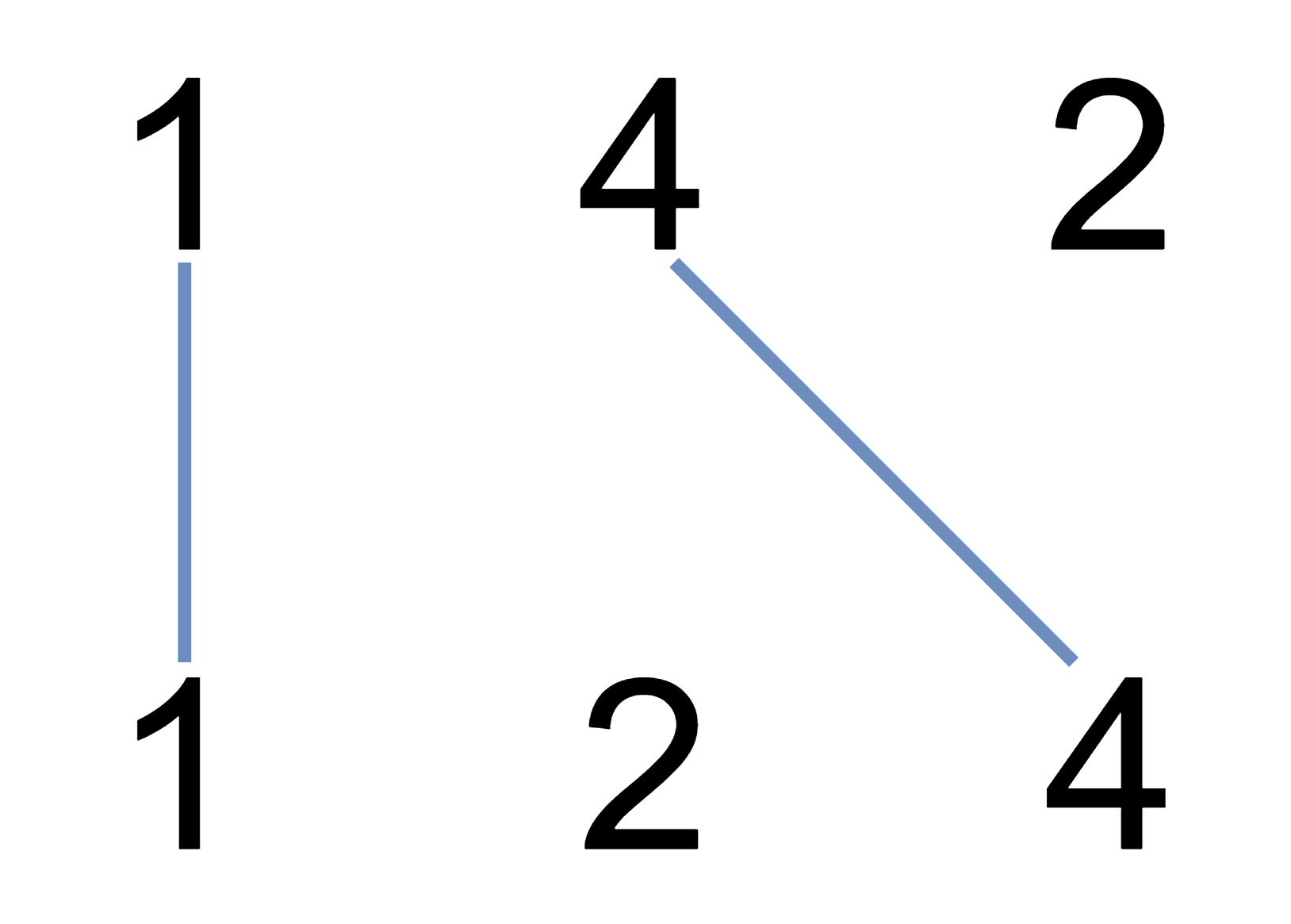1035. Uncrossed Lines

#### QUESTION:

We write the integers of A and B (in the order they are given) on two separate horizontal lines.

Now, we may draw connecting lines: a straight line connecting two numbers A[i] and B[j] such that:

1. A[i] == B[j];
2. The line we draw does not intersect any other connecting (non-horizontal) line. Note that a connecting lines cannot intersect even at the endpoints: each number can only belong to one connecting line.

Return the maximum number of connecting lines we can draw in this way.

Example 1:``````Input: A = [1,4,2], B = [1,2,4]
Output: 2
Explanation: We can draw 2 uncrossed lines as in the diagram.
We cannot draw 3 uncrossed lines, because the line from A=4 to B=4 will intersect the line from A=2 to B=2.
``````

Example 2:

``````Input: A = [2,5,1,2,5], B = [10,5,2,1,5,2]
Output: 3
``````

Example 3:

``````Input: A = [1,3,7,1,7,5], B = [1,9,2,5,1]
Output: 2
``````

Note:

``````1 <= A.length <= 500
1 <= B.length <= 500
1 <= A[i], B[i] <= 2000
``````

#### SOLUTION:

``````    public static int maxUncrossedLines(int[] A, int[] B) {
int m = A.length, n = B.length, dp[][] = new int[m + 1][n + 1];
for (int i = 1; i <= m; ++i)
for (int j = 1; j <= n; ++j)
if (A[i - 1] == B[j - 1])
dp[i][j] = 1 + dp[i - 1][j - 1];
else
dp[i][j] = Math.max(dp[i][j - 1], dp[i - 1][j]);
return dp[m][n];
}
``````